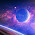Wednesday, January 30, 2013

Trust Bank Recruitment Test Answers of Management Trainee Officer 2011

1.thnk u.... but mone hosse ata guide theke copy mara hoise..

2.Is there any test paper of Eastern Bank Ltd?

1.3.i think ans of the Q.23 is: (a)48
from the eq. (p+1)/(q+1)=29/21 =>(p+1+q+1)/q+1=(29+21)/21 =>(p+q+2)/(q+1)=50/21
in this eq. if p+q=48 only then we find a integer value of q
Is there any other easy solution?....then plz reply

4.ans of 26 isn't (e)...it should be (a)0
Because integers are : ....-3,-2,-1,0,1,2,3....
so (-3)(-2)(-1)(0)=0

5.ans of (30) should be d.18
bcz Maximum total student could be (5*30)=150 so, 150*0.12=18